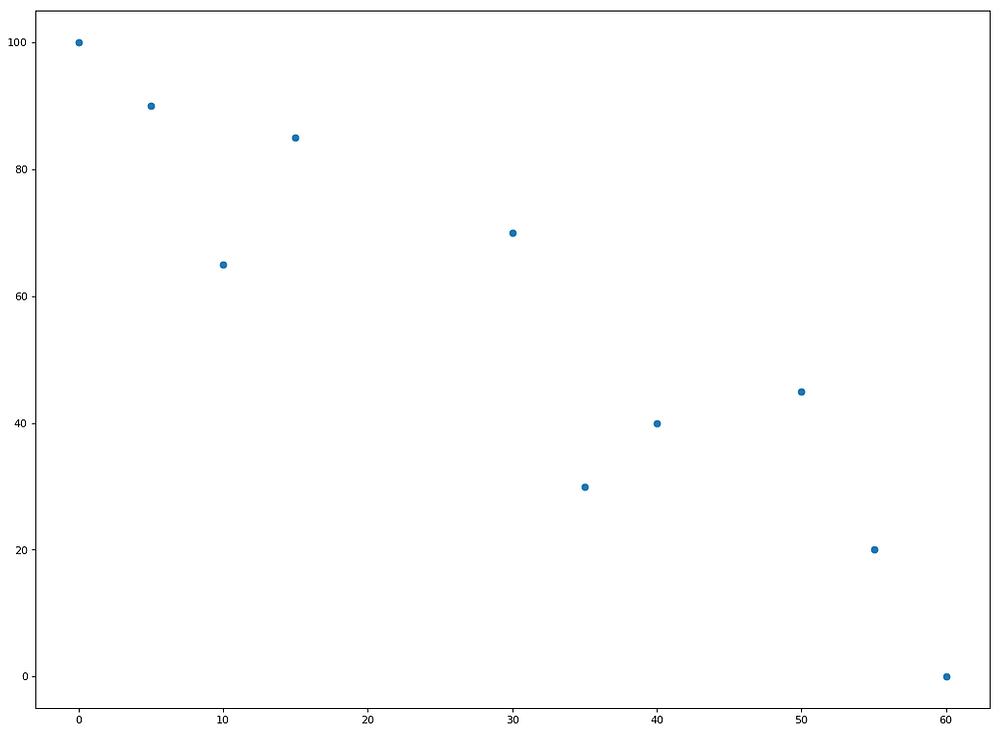Search
• Andrew Jones

# Creating and Plotting Cubic Splines in Python

A 'spline' is quite a generic term, essentially referring to applications of data interpolation or smoothing. It's a technique that can help you increase the frequency of your data, or to fill in missing time-series values.

In our example below, a dog is sniffing out a treat in the distance. At ten random points over the course of 60 seconds, the dog's collar is emits a statistic showing how far away the dog is from the treat.

From this data, we want to estimate the path the dog took to get to the treat. We can do this in Python!

Firstly, let's create our data, the times that the data was emitted and the subsequent distance statistics.

import numpy as np import matplotlib.pyplot as plt from scipy import interpolate

timestamp = (0,5,10,15,30,35,40,50,55,60) distance = (100,90,65,85,70,30,40,45,20,0)

data = np.array((timestamp,distance))

Let's plot what these points look like:

plt.plot(timestamp, distance, 'o') plt.show()Now, onto the spline creation. Note, that s=0 meaning that we're forcing the spline fit to pass through all the input points

tck,u = interpolate.splprep(data, s=0)

Below, we're creating the array of points that we want the new spline to pass through. Here, it will be 101 points (in order to fill all connections)

unew = np.arange(0, 1.01, 0.01)

Next, we apply the spline, giving us 101 values for time and for distance

out = interpolate.splev(unew, tck)

Let's now plot the new spline over our original data points!

plt.plot(out, out, color='orange') plt.plot(data[0,:], data[1,:], 'ob') plt.show()We now have an estimation as to the path the dog took, and therefore also the distance the dog was away from the treat at any point in the 60 seconds.

I hope you're able to make use of this technique!

Full Code

import numpy as np import matplotlib.pyplot as plt from scipy import interpolate

timestamp = (0,5,10,15,30,35,40,50,55,60) distance = (100,90,65,85,70,30,40,45,20,0)

plt.plot(timestamp, distance, 'o') plt.show()

data = np.array((timestamp,distance))

tck,u = interpolate.splprep(data, s=0) unew = np.arange(0, 1.01, 0.01) out = interpolate.splev(unew, tck)

plt.plot(out, out, color='orange') plt.plot(data[0,:], data[1,:], 'ob') plt.show()